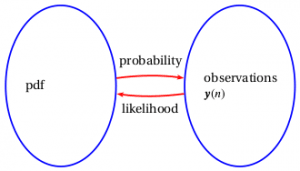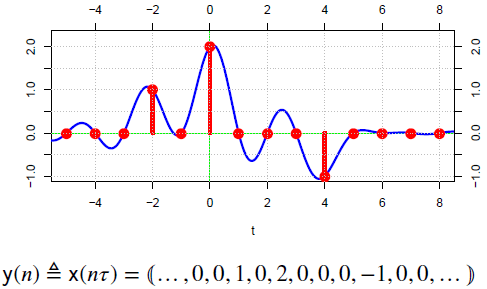# Introduction to Sampling

July 3, 2019

Sampling is a mathematical operation that transforms, or maps, a continuous waveform into a sequence of numbers. Typically, this involves taking measurements of the waveform at regular intervals and then structuring these measurements as a sequence of values.

Data sampling can be carried out using statistics or digital signal processing (DSP). Both yield useful insights. Statistical sampling provides a better understanding of the fundamental nature of the observed process. DSP transforms data into a sequence that can be further transformed by mathematical operations—e.g., filters.

### Statistics

In probability and statistics, a signal is a random variable defined in a probability space. A probability space consists of a sample space (𝛺), an event space, and a probability function. The signal determines the event that will occur in the observation space.Figure 1.1. The probability space and the observation space.

If we know the probability (P) of an event, we can estimate what we will observe during testing. This means the probability space predicts the observation space (see Figure 1.1.) However, if we don’t know the probability space, we can sample the observations and then infer what the probability space is likely to be.

Probability uses the probability space to determine the likely observation space. Statistics determine the likely probability space by using sample maps from the observation space.

#### Examples in Vibration Testing

Examples of estimated statistics based on collected samples are probability density, autocorrelation, cross-correlation, auto-spectral density, cross-spectral density, mean, and variance. In general, these quantities are not known, nor can they be. They can only be estimated from the samples provided by the mathematical operation of sampling.

### DSP

DSP uses operations that transform one sequence in a mathematical space into another sequence in the same space. In many real-world systems, DSP begins with sampling.

#### Examples in Vibration Testing

Examples of DSP operations on sequences include filtering, windowing, and sample rate conversion.Figure 1.2. Sampling a waveform.